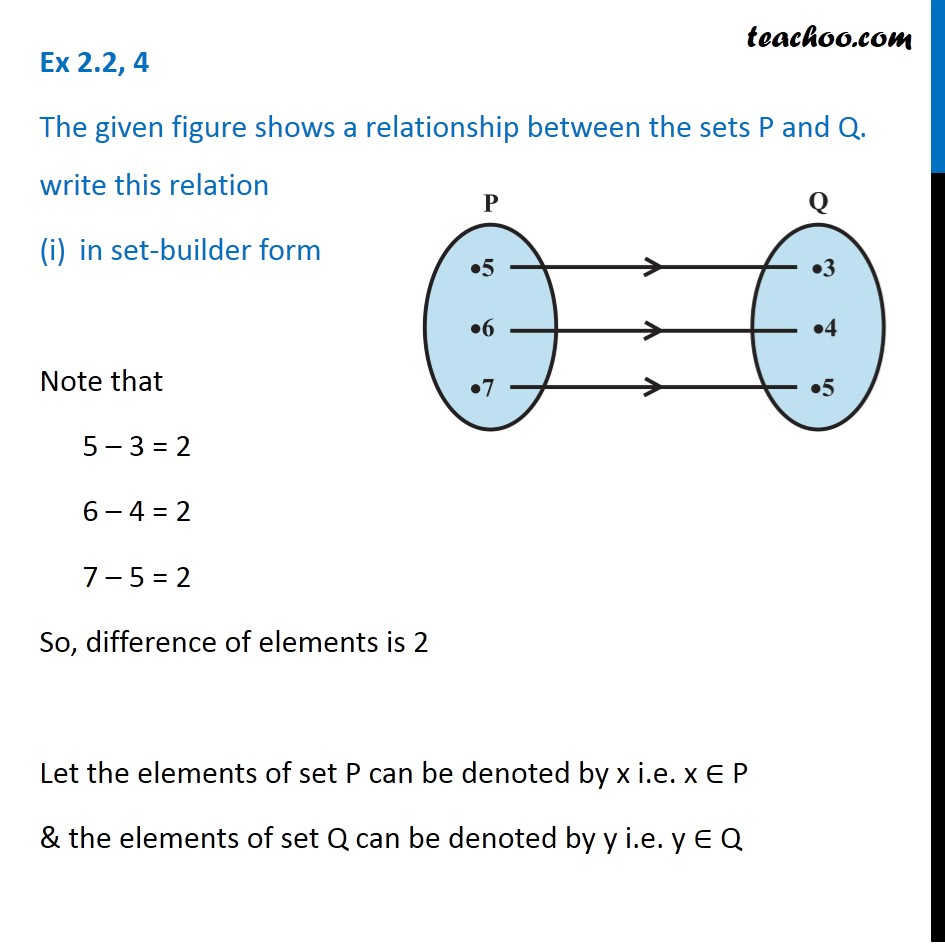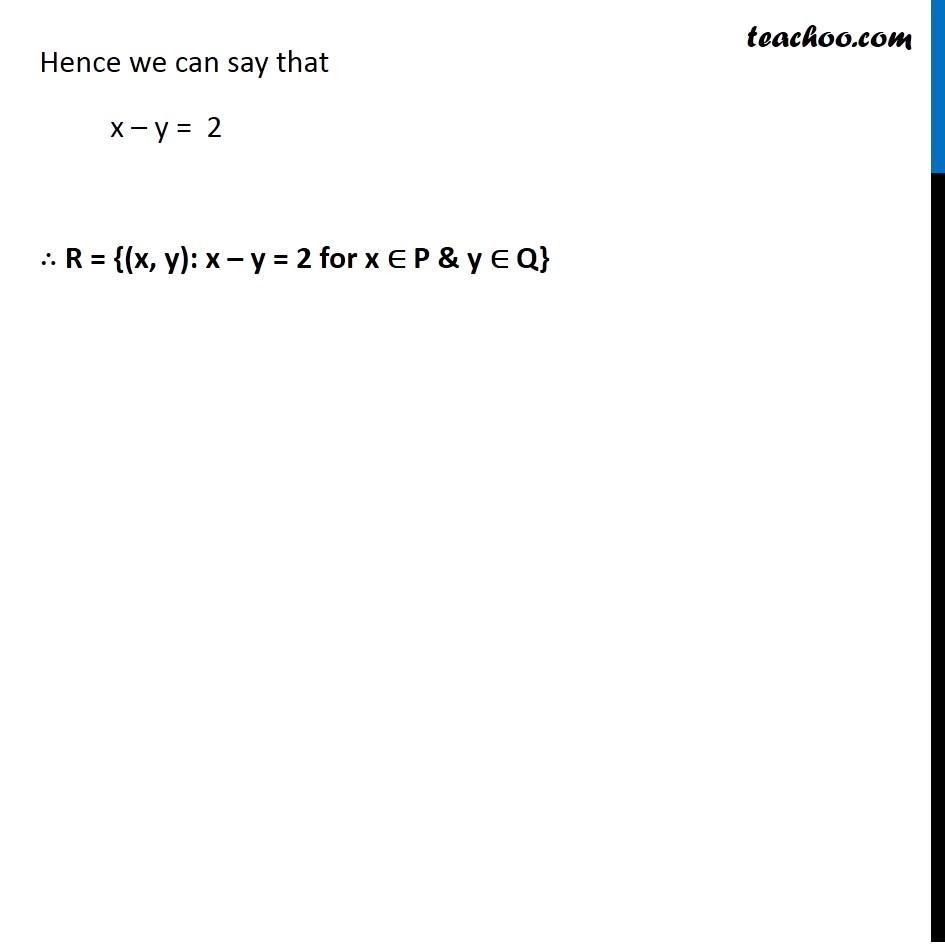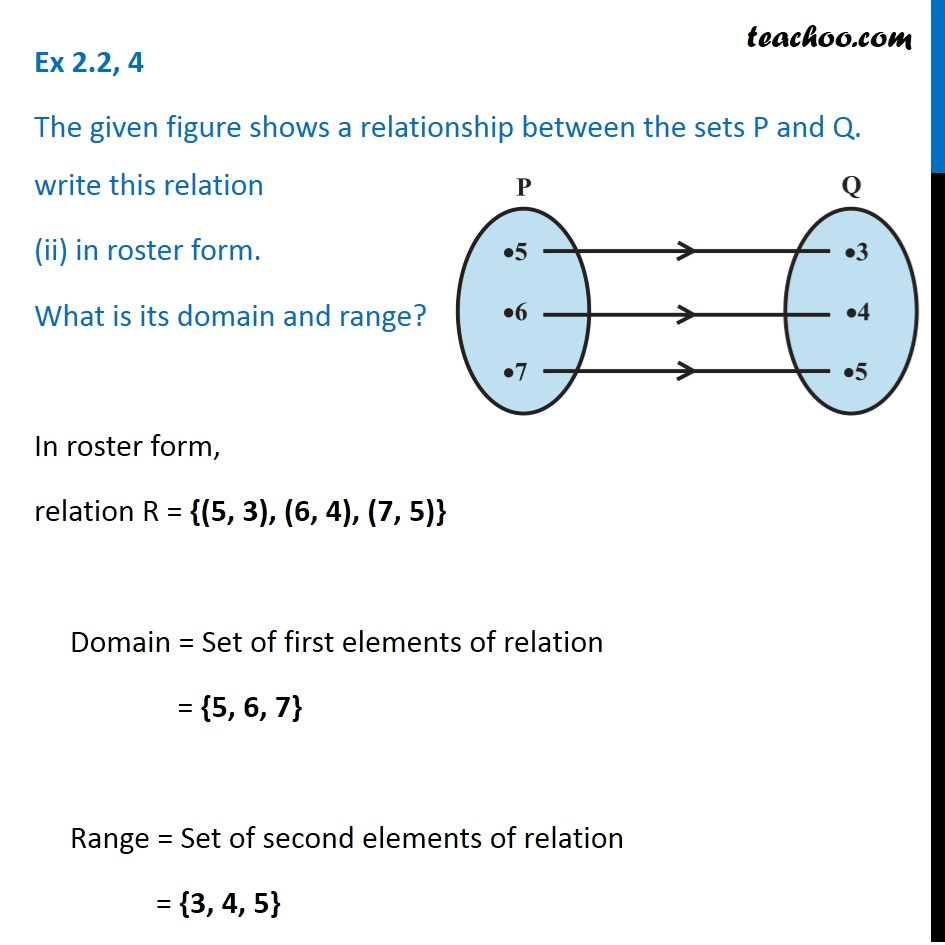Finding Relation - Arrow Depiction given

Chapter 2 Class 11 Relations and Functions
Concept wiseLearn in your speed, with individual attention - Teachoo Maths 1-on-1 Class

### Transcript

Ex 2.2, 4 The given figure shows a relationship between the sets P and Q. write this relation in set-builder form Note that 5 – 3 = 2 6 – 4 = 2 7 – 5 = 2 So, difference of elements is 2 Let the elements of set P can be denoted by x i.e. x ∈ P & the elements of set Q can be denoted by y i.e. y ∈ Q Hence we can say that x – y = 2 ∴ R = {(x, y): x – y = 2 for x ∈ P & y ∈ Q} Ex 2.2, 4 The given figure shows a relationship between the sets P and Q. write this relation (ii) in roster form. What is its domain and range? In roster form, relation R = {(5, 3), (6, 4), (7, 5)} Domain = Set of first elements of relation = {5, 6, 7} Range = Set of second elements of relation = {3, 4, 5}